Keep checking BNN for the breaking news updates, and discuss it on the forums or in the Bulbagarden Discord server.

Want to write an article? Go ahead! Have an opinion to share? An idea for a new column? Contact us!

# Crunching the numbers

Jump to navigationJump to search
Enhance your game with the power of math
• Tuesday, May 31, 2011

 This column has been written by Danielle Detering. It expresses the views of the columnist, not necessarily those of Bulbagarden networks.
Link to this article
 [url=https://bulbanews.bulbagarden.net/wiki/Crunching_the_numbers] Crunching the numbers[/url] Crunching the numbers

When I was a kid, I never brought what I learned in school into games. After an intense five hours of learning and one hour of homework (which, looking back, was a pretty sweet work schedule), the last thing I ever wanted to do was think about math. And thus, I typically spent most of my free time playing video games in an attempt to block the idea of learning out of my head.

Now, this is where Pokémon comes in. In Generation I, the power of a move wasn’t conveniently displayed in game, and I heard through the grapevine that when a Pokémon uses an attack of its same typing, it would be stronger. Naturally, I didn’t question it and always used this “hidden technique” throughout the game.

When Generation II started displaying the power of a move, I always used the move with the highest power whenever weaknesses and resistances didn’t make a difference. Makes sense right? But out of my curiosity, I tried to see if the super secret hidden technique still worked. The answer: not always. I was very confused. My strategy guide didn’t explain the phenomena, and my friends had no explanation for this occurrence. I did what any child of the nineties would do. I asked Jeeves.

And what did I find out? Holy Arceus! Stop the presses! Pokémon runs on math. Weaknesses and resistances? Special versus physical? STAB? It’s all just a giant game of numbers!

About a decade later, I’m a math major still crunching numbers for my videogames. However, almost anyone can use my favorite techniques of estimation. Here are some of the more useful tricks I know.

## Multiplying probabilities

Say we’re playing 2v2. On one side we have two fast Pokémon that know Blizzard and Rock Slide. On the other, we have two slow Pokémon that can survive one attack, but not two. As long as one of the slow Pokémon can survive, they can use a move to knock out the two fast ones. What are the chances that the two slow ones will survive? Assuming neutral conditions, Blizzard has a seventy percent (70% = .7) chance to hit and Rock Slide has a ninety percent (90% = .9) chance to hit. In probability theory, we can multiply two numbers together to see the chances of them both happening successfully. (For those of you that like technicalities, this only works as long as the two events being multiplied are independent of each other. Since Blizzard doesn’t affect Rock Slide in any way, this formula is perfectly legal in this situation.) So the chances that both Rock Slide and Blizzard will hit one of the opponent’s Pokémon is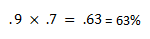.

So, there is a sixty-three percent chance that one of the slow Pokémon will be knocked out. Since this is only for one Pokémon, we need to multiply this number with itself to see what will happen to both Pokémon:. Therefore, there is about a forty percent chance that both the slow Pokémon will be knocked out. So what do these numbers tell us? Chances are, one of the slow ones will be knocked out, but one will survive to retaliate and redeem his or her fallen ally.

Note: In order to find the chances of something not happening, you can subtract the chances of something happening from one (in other words, the chances that none of the slow Pokémon would be knocked out is 1 - .3969 = .6031, or 60.31%. You can use this trick to, for example, find the chances that Blizzard hits only one Pokémon while Rock Slide only hits the other).

The ability to find the combined probability of two independent events occurring is a key skill in probability theory. It is the basis for many tools in the discipline. The following tip is a more complicated use of the same principle.

## Time calculations

While there is a 1/8192 chance to find a Shiny Pokémon, this does not mean that for every eight thousand one hundred ninety two Pokémon a person is guaranteed to find one Shiny. The actual chances of finding at least one Shiny in eight thousand one hundred ninety two Pokémon is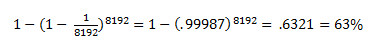.

To break down the above calculation, I first need to find the chances of not finding a Shiny in one encounter (.99987 = 99.987%). After that, I calculated the chances of not finding a Shiny in eight thousand one hundred ninety two encounters (.3678 = 36.78%). Since this is the chance of not finding a Shiny, I needed to subtract it from one, giving the result that there is about a sixty three percent chance to find at least one Shiny Pokémon in eight thousand one hundred ninety two encounters.

One may wonder how many encounters are need to have a ninety nine percent chance to find a Shiny. The basic calculation for that begins like so:, where x is going to be the number of encounters we’ll need. We can subtract one from both sides and multiply both sides by negative one. After simplifying, the expression turns into.

We need to use a function called a logarithm in order to find x. For those of you who don’t know what a logarithm is, Wikipedia probably gives a better explanation than I do. It is the inverse function to an exponent, which will allow us to get x by itself. For a simple online logarithm calculator, you can find one here.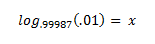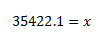This means I would have to run into thirty five thousand four hundred twenty two Pokémon in order to have a ninety nine percent chance to find at least one Shiny. If I can find fifty Pokémon in an hour, that would take me seven hundred and eight hours.

## Combinations

While very different from the technique used for time estimation, combinations are also based on multiplying probabilities to find the chances two or more events occur. Combinations are the number of different ways a number of objects can be arranged (order doesn’t matter). Seems trivial, but here’s an example: Say we’re playing around with Charmander, Charmeleon, and Charizard cards. We have one of each, and we keep on shuffling them together and drawing two. How many different ways can we draw two cards? The answer is three: Charmander and Charmeleon, Charmander and Charizard, and Charmeleon and Charizard. But say we add a Squirtle, a Wartortle, and Blastoise into the mix. It would take us way too much time to write down every combination of two cards, so instead, we’ll use the following formula: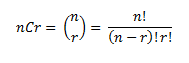, where n is the number of objects we have and r is the number of objects we’re choosing. In this case, we have six objects and we’re choosing two, so the formula would work out like this: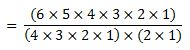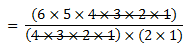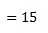Thus, there are fifteen ways we can choose two cards from our deck of six. This formula can not only tell us how many different ways we can draw so many cards from a deck, but we can also use it to estimate the chances of getting a specific card.

## Card draw estimation

Setting up quickly is important in the Trading Card Game (TCG), so it’s good to have a high probability to have the cards you need for the beginning of the game at the beginning of the game (I apologize for the obvious statement). Let’s say I desperately want to end my first turn with Spiritomb in the play area (either active or on the bench), so I put four of them in my deck. For the sake of simplicity, we’re going to ignore mulligans. So, the chances of getting Spiritomb in my opening hand is one minus the probability of having no Spritomb in my hand. Fifty six of the cards in my deck are not Spiritomb (60 – 4 = 56). Next, we’re going to break down the problem. If I draw just one card in my deck, the probability of not drawing a Spiritomb is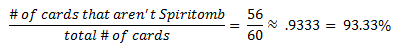.

If we subtract that number from one, that means we have under a 7% chance to draw a Spiritomb if we draw only one card. Luckily, we get to draw seven for our opening hand. This is where we get to use our earlier trick of multiplying probabilities together. We draw our next card. Since there is now one less card that isn’t Spiritomb in the deck and the total number of cards has decreased in the deck by one, the probability of the second card drawn not being Spiritomb is.

Now, we multiply these two numbers together to see what the probability that neither of the first two cards being drawn is Spiritomb. That is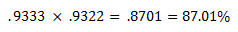. Those with a good eye for patterns can see where this is going. Let’s set up a table of probabilities for the first seven cards we draw.Multiplying the bottom row will tell us the probability of not having Spiritomb in our opening hand.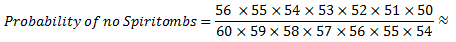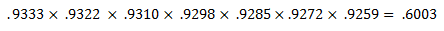Therefore, the probability of not having a Spiritomb in my opening hand is 60.03%, or conversely, I have a 39.97% chance of starting the game out with at least one of my four Spiritomb.

As mentioned earlier, combinations can be used to predict the same thing. First, we need to find the total number of ways seven cards can be drawn from a sixty card deck. So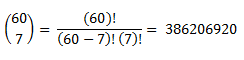. Second, we find the total number of ways we could draw seven cards that aren’t Spiritomb: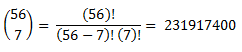. Finally, we divide the second number by the first:Note: It looks like there is a little bit of a rounding error. This is because the decimals I used in the earlier calculation were truncated so that they only had four digits. Therefore, 60.05% is probably a more accurate number.

But this is only the first step. This doesn’t tell me what my chances are of ending my first turn with a Spiritomb. One of two possibilities can happen from here: I could go first or I could go second. Let’s assume I got a basic Pokémon so we can continue the game. By going first, I don’t get to play any Trainers or Supporters, so all I can do is hope that the card I draw at the beginning of the turn is a Spiritomb. Since I don’t know what’s in my prizes at this point, as far as probability calculations go, I can still pretend I have fifty three cards left in my deck. Thus, I can do the same calculation I did before, but with drawing eight cards instead of seven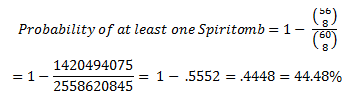.

However, if I go second, I can use Trainers and Supporters. Let’s say I have four Bebe’s Search and four Pokémon Collector along with my four Spiritomb. I can count both Bebe’s and Collector as a Spiritomb because I can easily use them to get one, ignoring the possibility that all four of my Spiritomb are in my prizes. Then the calculation turns into this: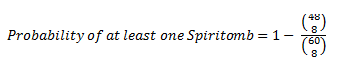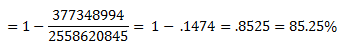And so, if I go second, I have at least an eighty-five percent chance to end the turn with a Spiritomb. Unfortunately, I can only go second fifty percent of the time. In order to find the total probability of ending my first turn with a Spiritomb, no matter whether I go first or second, I multiply both the chances of getting a Spiritomb if I go first and if I go second by one half to account for the fifty-fifty chance to go first. Then finally, I add them together to find the probability I have Spiritomb in the play area by the end of my first turn.Therefore, the probability I have at least one Spiritomb in the play area by the end of my first turn is just under sixty-five percent.

I hope this is helpful to all of you wishing to improve your game. Whether it be multiplying probabilities, calculating the time it takes to capture a Shiny Pokémon, estimating the probability of drawing a specific card, or using these or other tools to predict more phenomena in the world of Pokémon, these applications will not only improve a person’s understanding of the game, but (surprise!) they can be used to understand the real world better as well. But if there’s anything this game analyst can say is that experience, especially from losing, can be far better than any theory. So find a friend, pick up your DS or your deck, and go out there and have some fun!